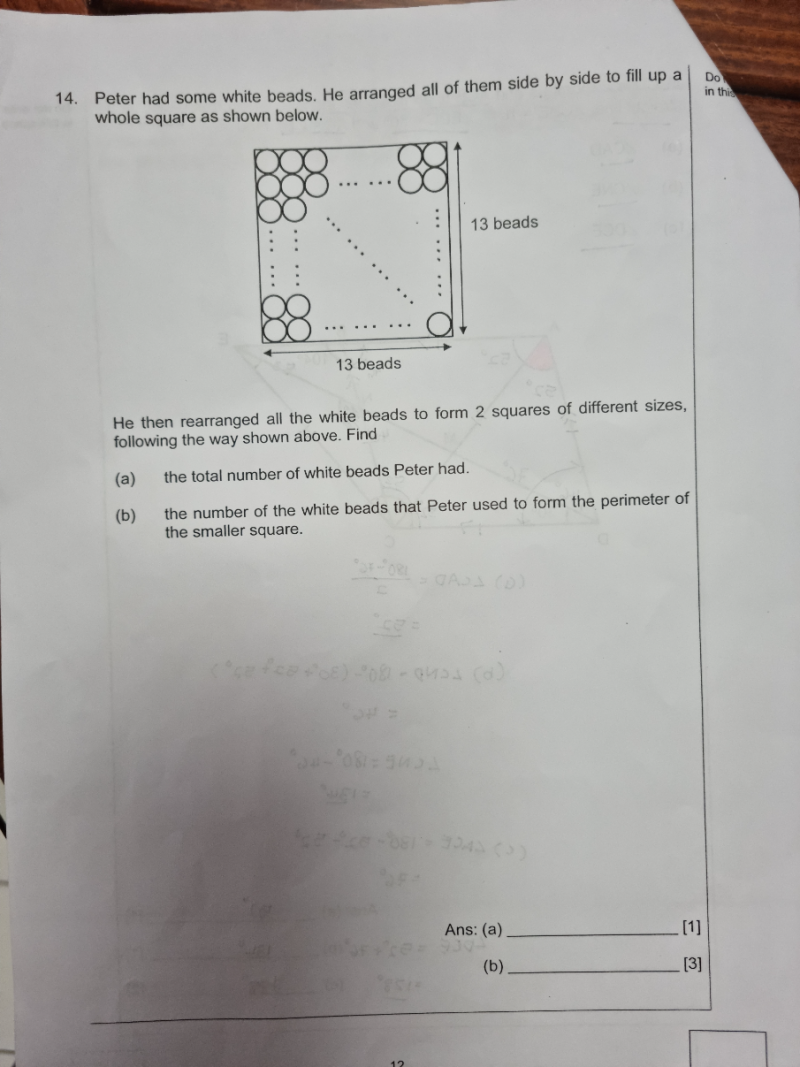# QuestionThank you.

(a) Total white beads Peter had = 13 x 13 = 169

(b) To form 2 squares, he would have one as 144 beads, then 169-144 = 25 to form the smaller square.

Each side of the smaller square is 5 white beads but since the corners share the same white bead,  then the perimeter of smaller square formed by the number of white beads would be  (5×4) – 4 = 16 beads

OOOOO

OOOOO

OOOOO

OOOOO

OOOOO